# Q5) Use shell method find the volume of the solid generated by revolving the curves y...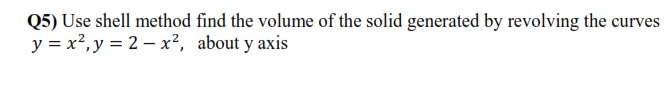Q5) Use shell method find the volume of the solid generated by revolving the curves y = x2, y = 2 - x2, about y axis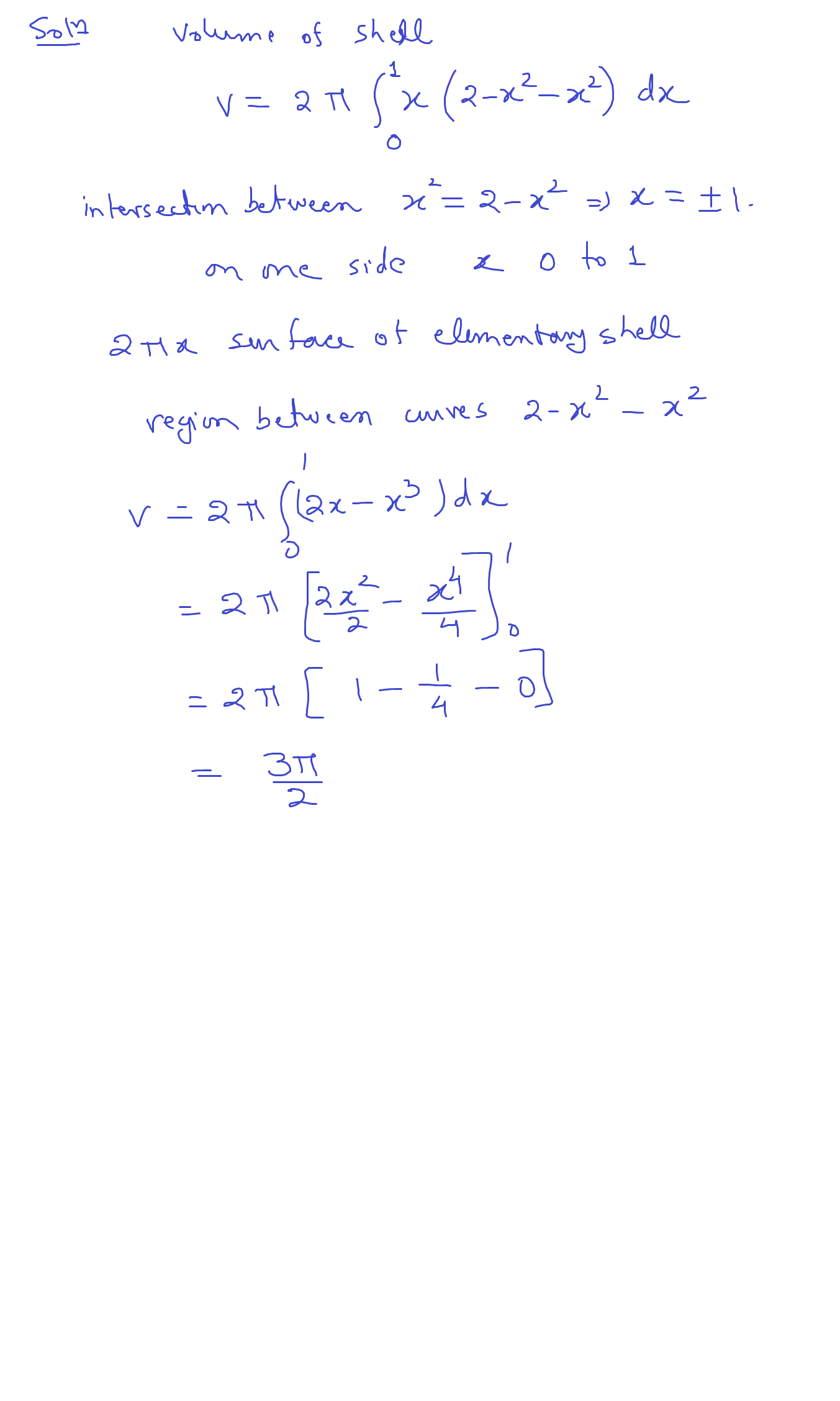!! PLEASE VOTE THUMBS UP !!

##### Add Answer of: Q5) Use shell method find the volume of the solid generated by revolving the curves y...
Similar Homework Help Questions
• ### Use the shell method to find the volume of the solid generated by revolving the region bounded by the line y 3x+10 and...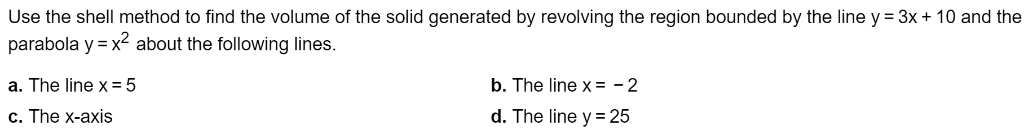Use the shell method to find the volume of the solid generated by revolving the region bounded by the line y 3x+10 and the parabola y x2 about the following lines. a. The line x 5 b. The line xE - 2 C. The x-axis d. The line y 25 Use the shell method to find the volume of the solid generated by revolving the region bounded by the line y 3x+10 and the parabola y x2 about the following...

• ### Use the shell method to find the volume of the solid generated by revolving the region...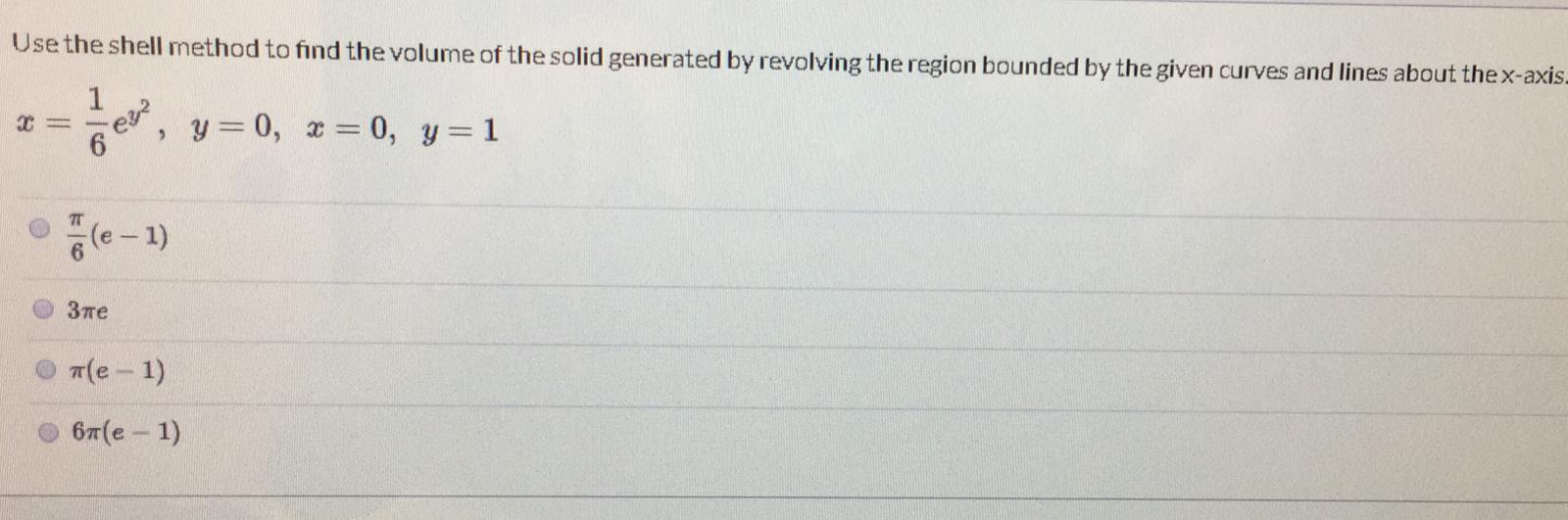Use the shell method to find the volume of the solid generated by revolving the region bounded by the given curves and lines about the x-axis- 1 = exe , y=0, x=0, y=1 6 (e-1) 3пе ale-1) 67(e - 1)

• ### Use the shell method to find the volume of the solid generated by revolving the shaded...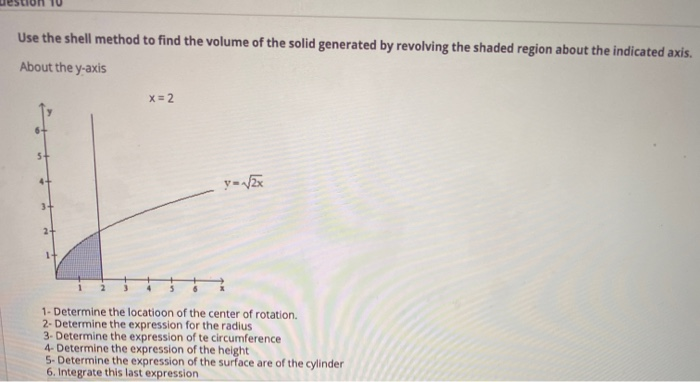Use the shell method to find the volume of the solid generated by revolving the shaded region about the indicated axis. About the y-axis x = 2 4 y-VEX 3+ 1- Determine the locatioon of the center of rotation. 2- Determine the expression for the radius 3. Determine the expression of te circumference 4-Determine the expression of the height 5. Determine the expression of the surface are of the cylinder 6. Integrate this last expression

• ### Please solve #13 and #17. In Exercises 13-16, use the shell method to set up and evaluate the integral that gives the volume of the solid generated hy revolving the plane region about the x-axis....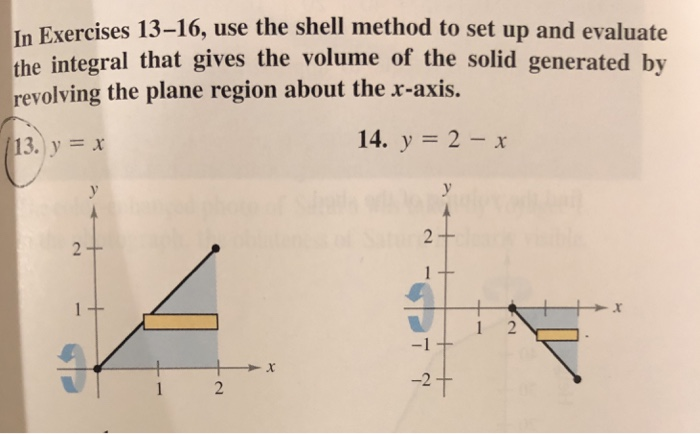Please solve #13 and #17. In Exercises 13-16, use the shell method to set up and evaluate the integral that gives the volume of the solid generated hy revolving the plane region about the x-axis. 14.,-2-х 13.) у х 12 -2十 In Exercises 17-20, use the shell method to find the volume of the solid generated by revolving the plane region about the indicated line. x2, y 4x x2, about the linex-4 y In Exercises 13-16, use the shell method...

• ### Use the shell method to find the volume of the solid generated by revolving the region bounded by the line y 5x +6 and...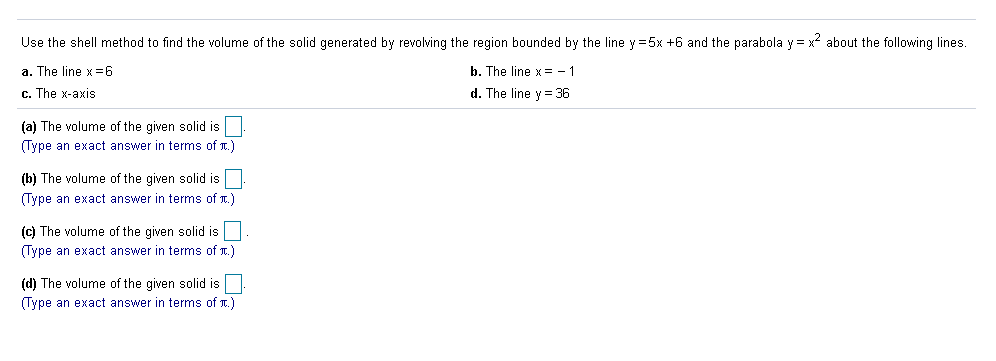Use the shell method to find the volume of the solid generated by revolving the region bounded by the line y 5x +6 and the parabola y = x about the following lines. a. The line x6 b. The line x= -1 c. The x-axis d. The line y36 (a) The volume of the given solid is (Type an exact answer in terms of .) (b) The volume of the given solid is (Type an exact answer in terms of...

• ### Use the shell method to find the volume of the solid generated by revolving the shaded region about the line y -1. O A....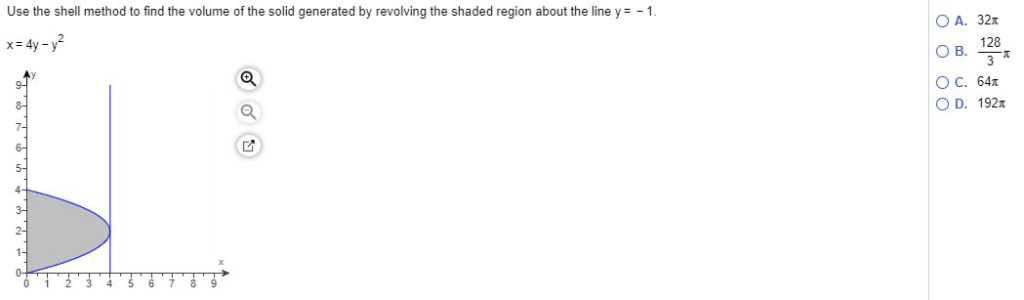Use the shell method to find the volume of the solid generated by revolving the shaded region about the line y -1. O A. 32x x 4y-2 128 O B. O C. 64x O D. 192x 8 7. 6- 5- 4 3 2- Use the shell method to find the volume of the solid generated by revolving the shaded region about the line y -1. O A. 32x x 4y-2 128 O B. O C. 64x O D. 192x 8...

• ### Step IS Test ebra Use the shell method to find the volume of the solid generated...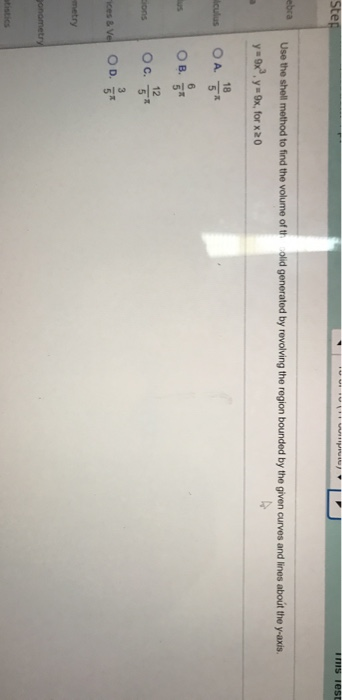Step IS Test ebra Use the shell method to find the volume of the solid generated by revolving the region bounded by the given curves and lines about the y-axis. y=9x, for x 20 yox? 18 diculus O A 5* 6 OB. 5* 12 sons Ос. 51 3 ces & Ve OD. 5* metry onometry

• ### Let R be the region bounded by the following curves. Use the method of your choice to find the volume of the solid gene...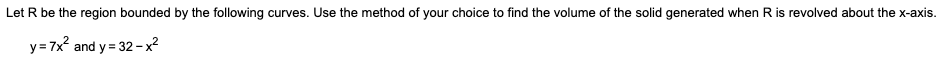Let R be the region bounded by the following curves. Use the method of your choice to find the volume of the solid generated when R is revolved about the x-axis. y=7x2 and y = 32-x2 Let R be the region bounded by the following curves. Use the shell method to find the volume of the solid generated when R is revolved about the x-axis. Let R be the region bounded by the following curves. Use the method of your...

• ### Use the disk or shell method to find the volume of the solid generated by revolving the region...Use the disk or shell method to find the volume of the solidgenerated by revolving the region bounded by the graphs of theequations about each given line.(a) the x-axis(b) the y-axis(c) the line x = 2

• ### Use the washer method to find the volume of the solid generated by revolving the region bounded...

Use the washer method to find the volume of the solid generated by revolving the region bounded by the parabola y=x^4 and the line y=8x about the y-axis.

Free Homework App